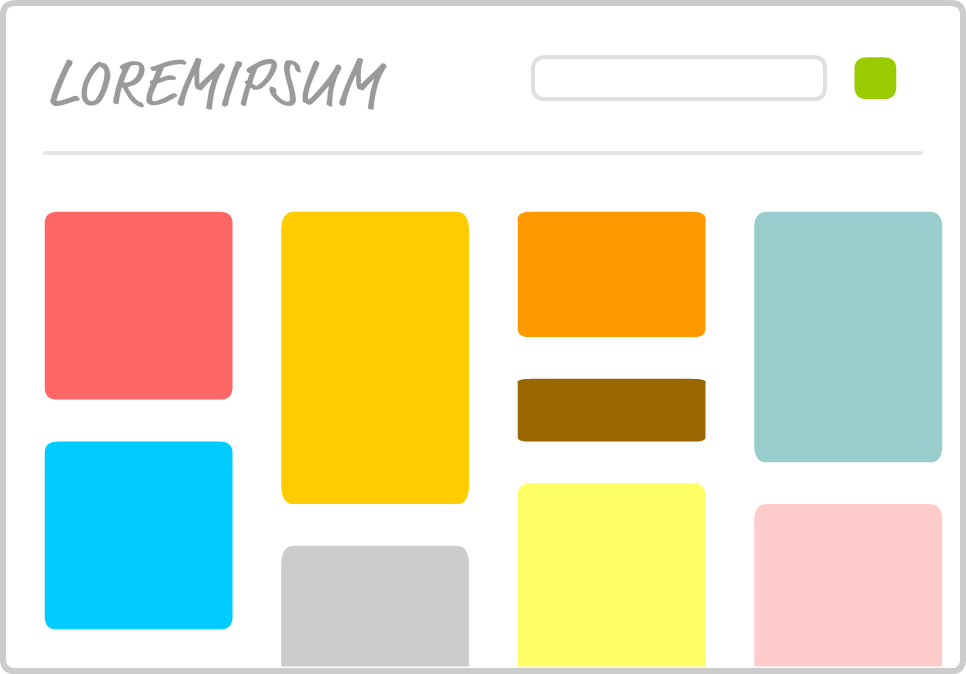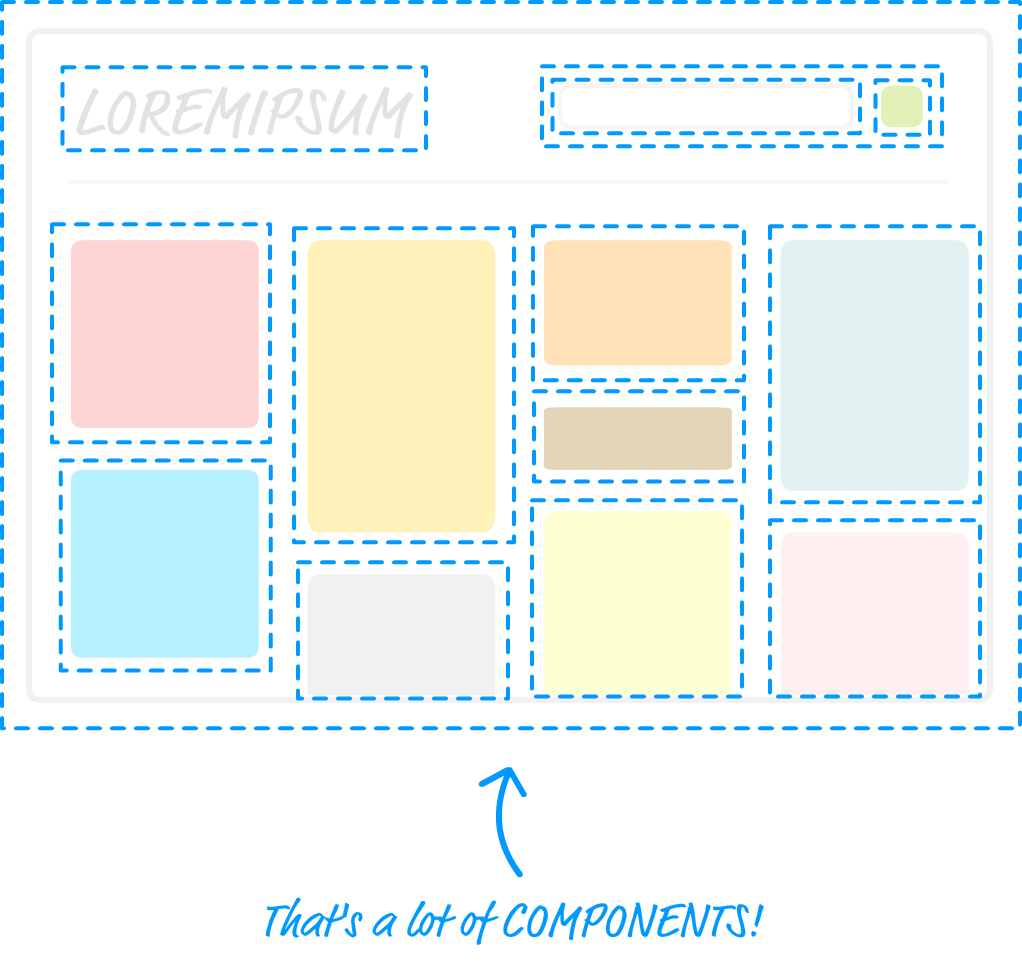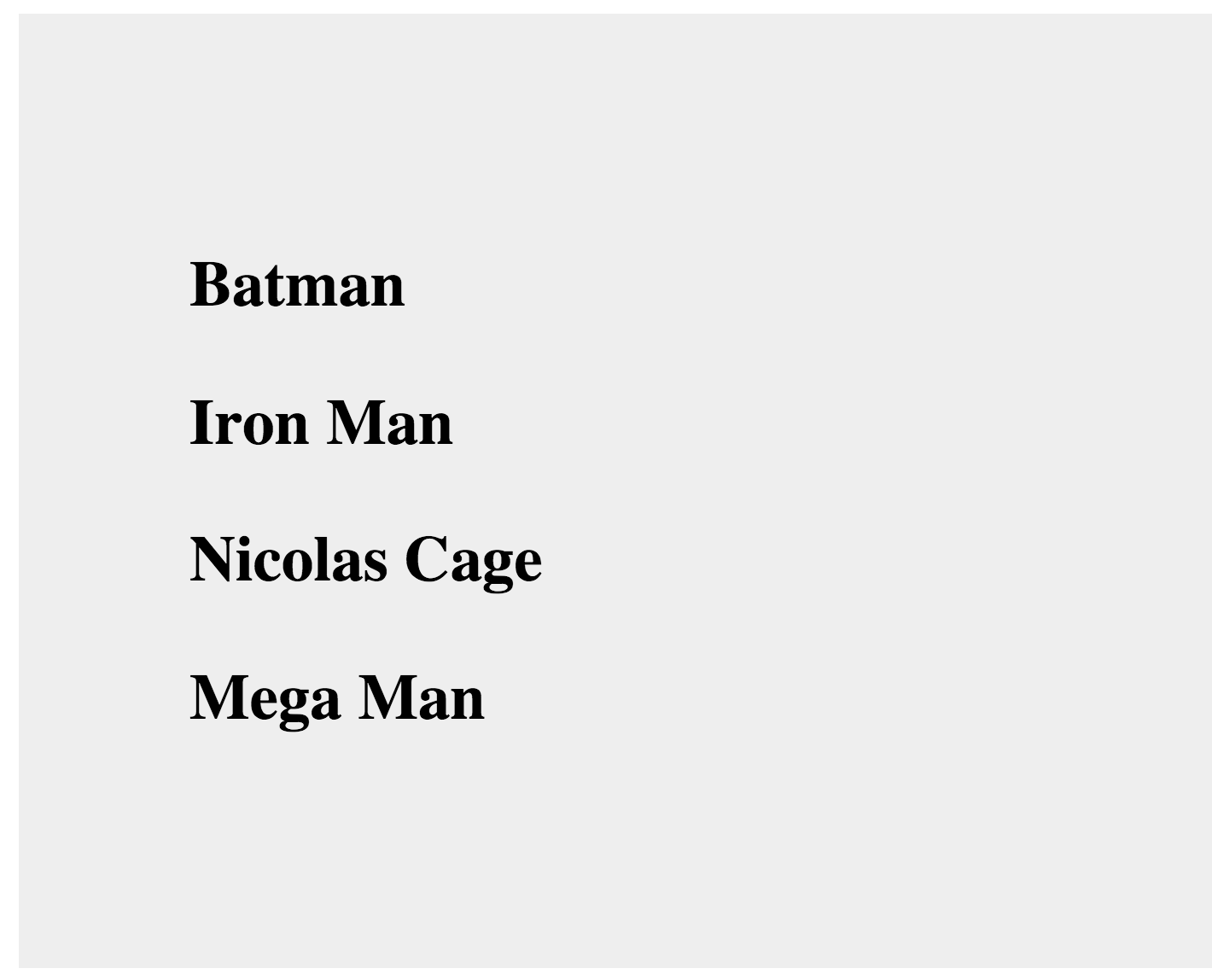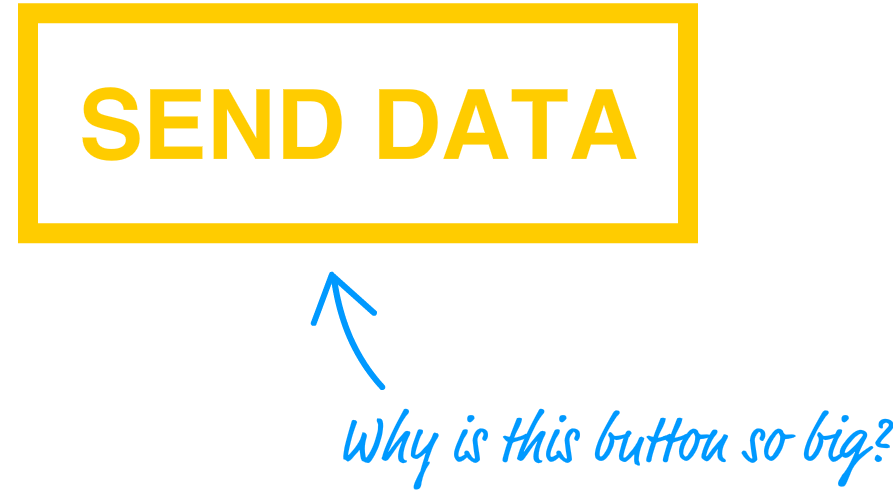# React 初学者教程 3: React 中的组件

• 网络埋伏纪事
• 6433 访问## 函数的快速回顾

``````var speed = 10;
var time = 5;

var speed1 = 85;
var time1 = 1.5;

var speed2 = 12;
var time2 = 9;

var speed3 = 42;
var time3 = 21;
``````

``````function getDistance(speed, time) {
var result = speed * time;
}
``````

``````getDistance(10, 5);
getDistance(85, 1.5);
getDistance(12, 9);
getDistance(42, 21);
``````

``````function formatDistance(distance) {
return distance + " km";
}

function getDistance(speed, time) {
var result = speed * time;
}
``````

## 改变处理 UI 的方式

``````var destination = document.querySelector("#container");

ReactDOM.render(
<h1>Batman</h1>,
destination
);
``````

``````var destination = document.querySelector("#container");

ReactDOM.render(
<div>
<h1>Batman</h1>
<h1>Iron Man</h1>
<h1>Nicolas Cage</h1>
<h1>Mega Man</h1>
</div>,
destination
);
``````

#### JSX 问题：输出多个元素**

``````var destination = document.querySelector("#container");
ReactDOM.render(
<h1>Batman</h1>
<h1>Iron Man</h1>
<h1>Nicolas Cage</h1>
<h1>Mega Man</h1>,
destination
);
``````

OK，现在我们有了四个 `h1` 元素，每个元素包含了一个英雄的名字。如果我们要把 `h1` 元素变为 `h3` 会是发生什么？我们可以手动修改这些元素如下：

``````var destination = document.querySelector("#container");

ReactDOM.render(
<div>
<h3>Batman</h3>
<h3>Iron Man</h3>
<h3>Nicolas Cage</h3>
<h3>Mega Man</h3>
</div>,
destination
);
````````````var destination = document.querySelector("#container");

ReactDOM.render(
<div>
<h3><i>Batman</i></h3>
<h3><i>Iron Man</i></h3>
<h3><i>Nicolas Cage</i></h3>
<h3><i>Mega Man</i></h3>
</div>,
destination
);
``````

``````var speed = 10;
var time = 5;

var speed1 = 85;
var time1 = 1.5;

var speed2 = 12;
var time2 = 9;

var speed3 = 42;
var time3 = 21;
``````

## 迎接 React 组件

OK，我们开始创建几个组件。首先创建一个空的 React 文档：

``````<!DOCTYPE html>
<html>

<title>React Components</title>
<script src="https://unpkg.com/react@15.3.2/dist/react.js"></script>
<script src="https://unpkg.com/react-dom@15.3.2/dist/react-dom.js"></script>
<script src="https://cdnjs.cloudflare.com/ajax/libs/babel-core/5.8.23/browser.min.js"></script>

<body>
<div id="container"></div>
<script type="text/babel">

</script>
</body>

</html>
``````

### 创建一个 “Hello, World!” 组件

``````ReactDOM.render(
<div>
<p>Hello, world!</p>
</div>,
document.querySelector("#container")
);
``````

``````var HelloWorld = React.createClass({

});

ReactDOM.render(
<div>
<p>Hello, world!</p>
</div>,
document.querySelector("#container")
);
``````

``````var HelloWorld = React.createClass({
render: function() {

}
});
``````

``````var HelloWorld = React.createClass({
render: function() {
return (
<p>你好，组件化的世界!</p>
);
}
});
``````

``````var HelloWorld = React.createClass({
render: function() {
return (
<p>你好，组件化的世界!</p>
);
}
});

ReactDOM.render(
<p>你好，组件化的世界!</p>,
document.querySelector("#container")
);
``````

``````ReactDOM.render(
<HelloWorld/>,
document.querySelector("#container")
);
``````

``````ReactDOM.render(
<div>
<HelloWorld/>
</div>,
document.querySelector("#container")
);
``````

``````ReactDOM.render(
<div>
<HelloWorld/>
<HelloWorld/>
<HelloWorld/>
<HelloWorld/>
<HelloWorld/>
<HelloWorld/>
</div>,
document.querySelector("#container")
);
``````

``````var HelloWorld = React.createClass({
render: function() {
return (
<p>Hello, world!</p>
);
}
});
``````

### 指定属性

``````function getDistance() {
}
``````

``````function getDistance(speed, time) {
var result = speed * time;
}
``````

#### 第一部分：修改组件定义

``````var HelloWorld = React.createClass({
render: function() {
return (
<p>Hello, {this.props.greetTarget}!</p>
);
}
});
``````

#### 第二部分：修改组件调用

``````ReactDOM.render(
<div>
<HelloWorld greetTarget="Batman"/>
<HelloWorld greetTarget="Iron Man"/>
<HelloWorld greetTarget="Nicolas Cage"/>
<HelloWorld greetTarget="Mega Man"/>
<HelloWorld greetTarget="Bono"/>
<HelloWorld greetTarget="Catwoman"/>
</div>,
document.querySelector("#container")
);
``````

### 处理子元素

``````<CleverComponent foo="bar">
<p>Something!</p>
</CleverComponent>
``````

``````var Buttonify = React.createClass({
render: function() {
return (
<div>
<button type={this.props.behavior}>{this.props.children}</button>
</div>
);
}
});
``````

``````ReactDOM.render(
<div>
<Buttonify behavior="Submit">SEND DATA</Buttonify>
</div>,
document.querySelector("#container")
);
``````## 总结